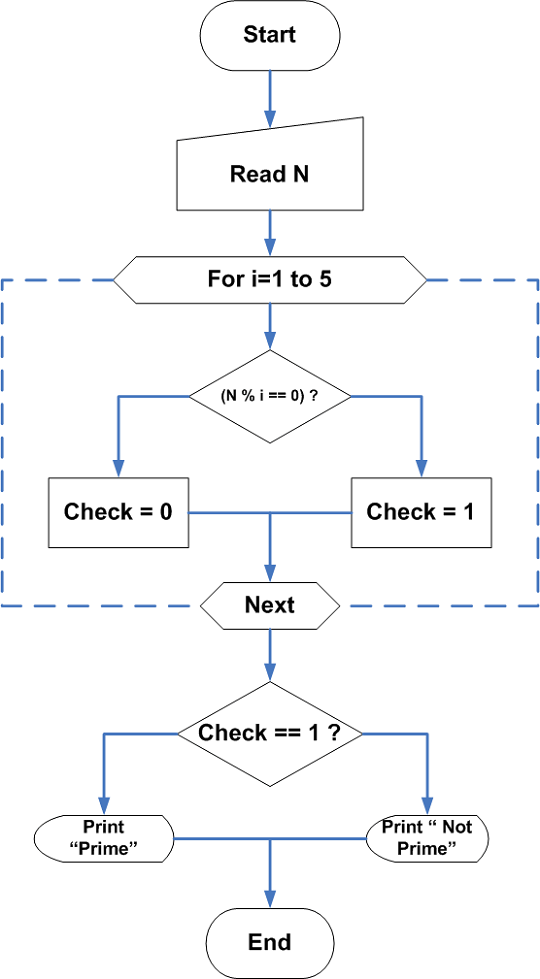# C Program To Check If A Number Is Prime Or Not

This program receives an input number from user and check if the number is prime number or not.The number is divided by 2, 3 and 5 to see if the remainder of the division is 0. If the remainder is zero, then the number is not prime otherwise, it is prime number.

We compiled the program using Dev-C++ compiler version 4.9.9.2 installed on a Windows 7 64-bit system. You may use other compilers such as Turbo C++ 3.0 after modifying the program source code. This is necessary to avoid any error in the program.

We recommend you to learn following C programming concepts before you begin with the example program.

Contents

### Problem Definition

Prime numbers are very important topic in mathematics. It is usually easy to find the small prime numbers, but very difficult to identify a prime number, when the numbers grow larger.We want to write a program to test whether a given number is a prime number or not.

What is a Prime number ?

Any positive number greater than 1 which is divisible only by itself and number one is called a Prime number, all other numbers are Composite numbers.

For example

63 = 3 \times 21

The number$63$ has two factors$3$ and$21$, therefore it is a composite number.

73 = 73 \times 1

The factors of number$73$ are$73$ itself and$1$, therefore,$73$ is a prime number.

How do we process the given input number?

Here are the steps to process the input number in the program.

Step 1 – Get the number,$N$.

Step 2 – Check if the number,$N$ is divisible by$2, 3,$ or$5$.

Step 3 – If the number,$N$ is divisible, then the$remainder = = 0$.

Step 4 – If number is divisible, then$check = 0$, else$check = 1$.

Step 5 – If$check = = 1$, number is a prime number

Step 6 – Print the output

Step 7 – End the program

### Flowchart – Program to check a Prime numberFlowchart- C Program to Check a Number if Prime Number or Not

### Program Code – Prime Number Check

/*Program to Check if the number is Prime or not */
#include <stdio.h>
#include <stdlib.h>
main()
{
int i,check,num; int a,b,c;
/* Get the number */
printf("Enter a number:");
scanf("%d",&num);
/* check if the number is divisible by 2,3 or 5*/
for( i=1; i<=5;i++)
{
if(num % i == 0)
{
check = 0;
}
else
{
check = 1;
}
}

/*print the result */
if(check == 1)
{
for(i=0;i<35;i++)
printf("_");printf("\n\n");
printf("The Number %d is a Prime Number\n\n",num);
for(i=0;i<35;i++)
printf("_");printf("\n\n");
}
else
{
for(i=0;i<35;i++)
printf("_");printf("\n\n");
printf("The Number %d is not a Prime Number\n\n",num);
for(i=0;i<35;i++)
printf("_");printf("\n\n");
}
getch();
return 0;
}

The for loop in the above source code continuously divides the given number to verify if remainder becomes a zero. If not then variable check is 1.

The program performs a second test in which if the value of variable check is$0$, it prints “Number is not a prime number” and if the value of variable check is$1$, prints “The Number is a prime number”.

### Output

         Enter a number:71
---------------------------------------
The Number 71 is a Prime Number
---------------------------------------